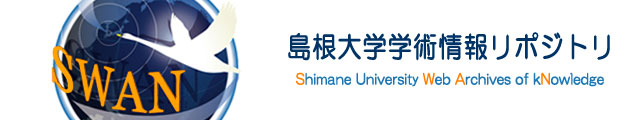言語 英語 著者 Ishibashi, Kazuki Department of Electronic Control Engineering, National institute of Technology, Hiroshima College, 内容記述（抄録等） As is well known, Mathieu’s equation is a representative of mathematical models describingparametric excitation phenomena. This paper deals with the oscillation problem forMathieu’s equation with two frequencies. The ratio of these two frequencies is not necessarilya rational number. When the ratio is an irrational number, the coefficient of Mathieu’sequation is is quasi-periodic, but not periodic. For this reason, the basic knowledgefor linear periodic systems such as Floquet theory is not useful. Whether all solutions ofMathieu’s equation oscillate or not is determined by parameters and frequencies. Our resultsprovide parametric conditions to guarantee that all solutions are nonoscillatory. Theadvantage of the obtained parametric conditions is that it can be easily checked. Parametricnonoscillation region is drawn to understand these results easily. Finally, severalsimulations are carried out to clarify the remaining problems. 主題 Nonoscillation Parametric excitation Mathieu’s equation Frequencies Quasi-periodic 掲載誌名 Applied Mathematics and Computation 巻 346 開始ページ 491 終了ページ 499 ISSN 0096-3003 発行日 2019-04 DOI 10.1016/j.amc.2018.10.072 出版者 Elsevier 資料タイプ 学術雑誌論文 ファイル形式 PDF 著者版/出版社版 著者版 部局 総合理工学部 数理科学科